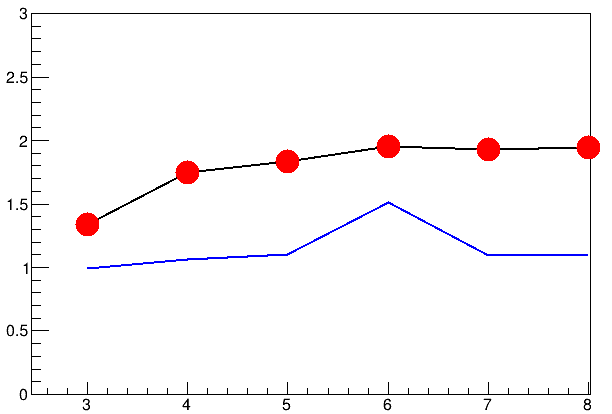# Drawing two graphs in one canvas properly with ROOT

I’m trying to plot two graphs in one canvas using Cern ROOT. When I use `gr -> Draw("ap")` and `gr2 -> Draw("p1 same")`, the lines of the plots are not visible, only the markers are visible. When I use `gr1 -> Draw("")` and `gr2 -> Draw("same")`, the first plot is visible with line and marker but the second plot is only visible with line and not markers.

I want to see both of their lines and markers. How can I do it? My code:

``````graph_one -> GetXaxis() -> SetTitle(" x ");
graph_one -> GetYaxis() -> SetTitle(" y ");
graph_one -> GetXaxis() -> SetRangeUser(0,8);
graph_one -> GetYaxis() -> SetRangeUser (0,3);
graph_one -> SetLineWidth(2);
graph_one -> SetLineColor(kBlack);
graph_one -> SetMarkerStyle(8);
graph_one -> SetMarkerSize(3);
graph_one -> SetMarkerColor(kRed);

graph_two -> SetLineWidth(2);
graph_two -> SetMarkerStyle(8);
graph_two -> SetMarkerSize(3);
graph_two -> SetLineColor(kBlue);
graph_two -> SetMarkerColor(kBlack);

graph_one -> Draw("");
graph_two -> Draw("same");
``````ROOT Version: 6.23/01
Platform: Linux
Compiler: Not Provided